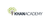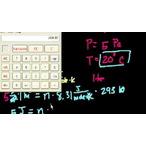# Class 11 Physics (India): Thermodynamics part 5: Molar ideal gas law problem

Product type
Total time

## Class 11 Physics (India): Thermodynamics part 5: Molar ideal gas law problemKhan Academy

Need more information? Get more details on the site of the provider.### Description

Sal uses the molar version of the ideal gas law to solve for the number of moles in a gas. He also shows how to convert this answer into number of molecules using Avogadro's number.

## Class 11 Physics (India)

Let's learn, practice, and master topics of class 11 physics (NCERT) starting with kinematics and then moving to dynamics with Newton's laws of motion, work, energy, and power. Let's then use these as the foundation to learn about centre of mass, rotational motion, gravitation, solids, fluids, thermodynamics, and oscillations and waves.

## Temperature, kinetic theory, and the ideal gas law

In these videos and articles you'll learn about the Celsius and Kelvin temperature scales. The definiti…

There are no frequently asked questions yet. If you have any more questions or need help, contact our customer service.

Didn't find what you were looking for? See also: Oil & Gas, Law (General), General Management, Software / System Engineering, and Health Management.

Sal uses the molar version of the ideal gas law to solve for the number of moles in a gas. He also shows how to convert this answer into number of molecules using Avogadro's number.

## Class 11 Physics (India)

Let's learn, practice, and master topics of class 11 physics (NCERT) starting with kinematics and then moving to dynamics with Newton's laws of motion, work, energy, and power. Let's then use these as the foundation to learn about centre of mass, rotational motion, gravitation, solids, fluids, thermodynamics, and oscillations and waves.

## Temperature, kinetic theory, and the ideal gas law

In these videos and articles you'll learn about the Celsius and Kelvin temperature scales. The definition of a mole of a substance will be given. You'll also learn how the ideal gas law relates the pressure, volume, and temperature of a gas. Lastly, you'll learn how the Maxwell-Boltzmann distribution gives the probability of finding a gas molecule moving at a specific speed.

## Topic: Science

Learn about all the sciences, from physics, chemistry and biology, to cosmology and astronomy, across hundreds of videos, articles and practice questions.

There are no reviews yet.